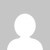# Version Space and List-Then-Eliminate Algorithm

[wptelegram-join-channel]

## Consistent Hypothesis, Version Space and List-Then-Eliminate Algorithm

An hypothesis h is said to be consistent hypothesis with a set of training examples D iff  h(x) = c(x) for each example in D,

### For Example:

h1 = (?, ?, No, ?, Many) – Consistent Hypothesis as it is consistent with all the training examples

h2 = (?, ?, No, ?, ?) – Inconsistent Hypothesis as it is inconsistent with first training example

### Version Space

The version space VSH,Dis the subset of the hypothesis from H consistent with the training example in D,

## List-Then-Eliminate algorithm

#### Steps in List-Then-Eliminate Algorithm

1. VersionSpace = a list containing every hypothesis in H

2. For each training example, <a(x), c(x)> Remove from VersionSpace any hypothesis h for which h(x) != c(x)

3. Output the list of hypotheses in VersionSpace.

### Example:

F1  – > A, B

F2  – > X, Y

Here F1 and F2 are two features (attributes) with two possible values for each feature or attribute.

Instance Space: (A, X), (A, Y), (B, X), (B, Y) – 4 Examples

Hypothesis Space: (A, X), (A, Y), (A, ø), (A, ?), (B, X), (B, Y), (B, ø), (B, ?), (ø, X), (ø, Y), (ø, ø), (ø, ?), (?, X), (?, Y), (?, ø), (?, ?)  – 16 Hypothesis

Semantically Distinct Hypothesis : (A, X), (A, Y), (A, ?), (B, X), (B, Y), (B, ?), (?, X), (?, Y (?, ?), (ø, ø) – 10

### List-Then-Eliminate Algorithm Steps

Version Space: (A, X), (A, Y), (A, ?), (B, X), (B, Y), (B, ?), (?, X), (?, Y) (?, ?), (ø, ø), •Training Instances

F1  F2  Target

A  X      Yes

A  Y      Yes

Consistent Hypothesis are (Version Space):   (A, ?), (?, ?)

### Problems with List-Then-Eliminate Algorithm

The hypothesis space must be finite

Enumeration of all the hypothesis, rather inefficient

## Summary

This tutorial discusses the Consistent Hypothesis, Version Space, and List-Then-Eliminate Algorithm in Machine Learning. If you like the tutorial share with your friends. Like the Facebook page for regular updates and YouTube channel for video tutorials.

### 2 thoughts on “Version Space and List-Then-Eliminate Algorithm”

1.